Next: Higher-order cumulants Up: One-unit contrast functions Previous: One-unit contrast functions

### Negentropy

A most natural information-theoretic one-unit contrast function is negentropy. From Eq. (15), one is tempted to conclude that the independent components correspond to directions in which the differential entropy of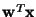is minimized. This turns out to be roughly the case. However, a modification has to be made, since differential entropy is not invariant for scale transformations. To obtain a linearly (and, in fact, affinely) invariant version of entropy, one defines the negentropy J as follows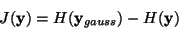(22)

where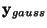is a Gaussian random vector of the same covariance matrix as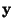. Negentropy, or negative normalized entropy, is always non-negative, and is zero if and only ifhas a Gaussian distribution .

The usefulness of this definition can be seen when mutual information is expressed using negentropy, giving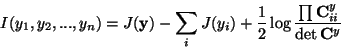(23)

where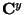is the covariance matrix of, and the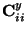are its diagonal elements. If the yi are uncorrelated, the third term is 0, and we thus obtain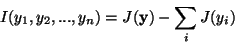(24)

Because negentropy is invariant for linear transformations , it is now obvious that finding maximum negentropy directions, i.e., directions where the elements of the sum J(yi)are maximized, is equivalent to finding a representation in which mutual information is minimized. The use of negentropy shows clearly the connection between ICA and projection pursuit. Using differential entropy as a projection pursuit index, as has been suggested in [57,78], amounts to finding directions in which negentropy is maximized.

Unfortunately, the reservations made with respect to mutual information are also valid here. The estimation of negentropy is difficult, and therefore this contrast function remains mainly a theoretical one. As in the multi-unit case, negentropy can be approximated by higher-order cumulants, for example as follows :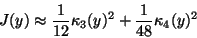(25)

where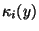is the i-th order cumulant of y. The random variable y is assumed to be of zero mean and unit variance. However, the validity of such approximations may be rather limited. In , it was argued that cumulant-based approximations of negentropy are inaccurate, and in many cases too sensitive to outliers. New approximations of negentropy were therefore introduced. In the simplest case, these new approximations are of the form: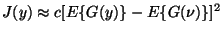(26)

where G is practically any non-quadratic function, c is an irrelevant constant, and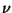is a Gaussian variable of zero mean and unit variance (i.e., standardized). For the practical choice of G, see below. In , these approximations were shown to be better than the cumulant-based ones in several respects.

Actually, the two approximations of negentropy discussed above are interesting as one-unit contrast functions in their own right, as will be discussed next.Next: Higher-order cumulants Up: One-unit contrast functions Previous: One-unit contrast functions
Aapo Hyvarinen
1999-04-23